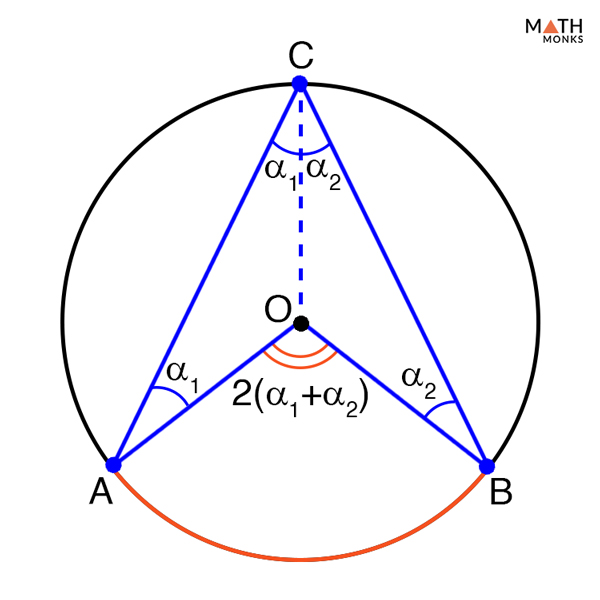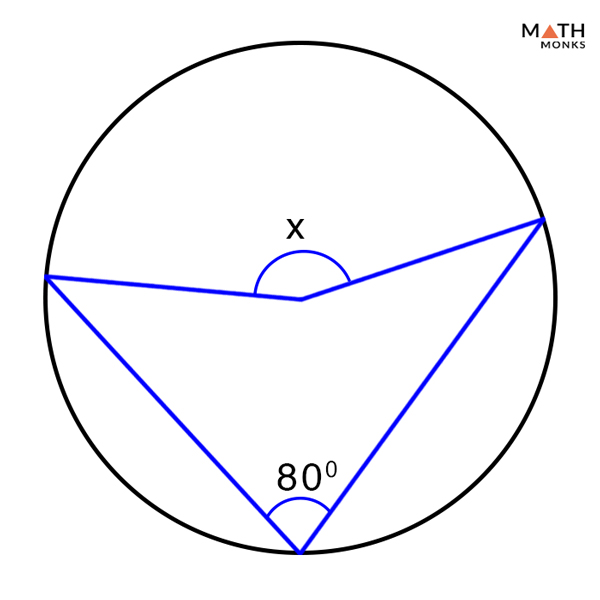# Central Angle

## What is a Central Angle

A central angle is an angle formed between two different radii of a circle. They are angle subtended to the center of a circle from two different points. Thus the vertex of the central angle will always be the center point of a circle.

The above figure shows a circle with center O having a central angle, ∠AOB. The two arms forming the angle are OA and OB where the two points A and B can be isolated points or they could be the end points of an arc and chord.

## Central Angle TheoremProve Central Angle Theorem

To prove:

∠AOB = 2∠ACB

Proof:

Let ∠AOB be the central angle, and ∠ACB the inscribed angle of the circle.
Since lines OC, OA, and OB are the radius of the circle and are of the same length,
Hence,
△COA and △COB are both isosceles triangles.
Let, ∠ACO = α1 and ∠BCO = α2
Then we can write,
∠CAO = α1 and ∠CBO = α2 (Isosceles triangles)
Again,
∠COA = 180° – 2α1….. (1)(Sum of the angles in a triangle is 180°)
Similarly,
∠COB = 180° – 2α2….. (2)
Since, all angles at center O add up to 360°,
∠AOB + ∠COA + ∠COB = 360°
∠AOB = 360° – (∠COA + ∠COB)…….. (3)
Substituting the value of (1) and (2) in (3) we get,
∠AOB = 360° -(180° – 2α1) – (180° – 2α2)
∠AOB = 360° – 180° + 2α1 – 180° + 2α2
∠AOB = 2α1 + 2α2
Thus,
∠AOB =2∠ACB
Hence Proved

## Formulas

### How to Find Central Angle

Central angle of a circle can be determined if its corresponding inscribed angle is known by using the formula derived from the central angle theorem given below:

Central angle = 2 x Inscribed angle

Alternative formula:

The central angle of a circle can also be determined if the arc length and the radius forming the angle is know, using the following formula:

Central angle = Arc length x 360°/2πr

Let us solve some problems to understand the concepts better.Solve the missing angle x in the diagram given below.

Solution:

As we know,
According to the central angle theorem the measure of the central angle in twice the measure of the central angle,
Given,
Inscribed angle = 80°
Thus,
Inscribed angle = 2 x 80° =160°

Find the central angle, where the arc length is 30 cm and the length of the radius is 15 cm. Given π = 3.14.

Solution:

As we know,
Central angle = Arc length x 360°/2πr, here arc length = 30 cm, r = 15 cm
= 30 x 360°/2 x 3.14 x 15
= 10800°/94.2
= 114.64°

If the central angle of a circle is 88° and the arc length formed is 28 cm. Find the radius of the circle. Given π = 3.14.

Solution:

As we know,
Central angle = Arc length x 360°/2πr, here central angle = 88°arc length = 28 cm
88° = 28 x 360°/ 2 x 3.14 x r
r = 28 x 360°/2 x 3.14 x 88
= 10080°/552.64
= 18.23 cm